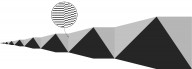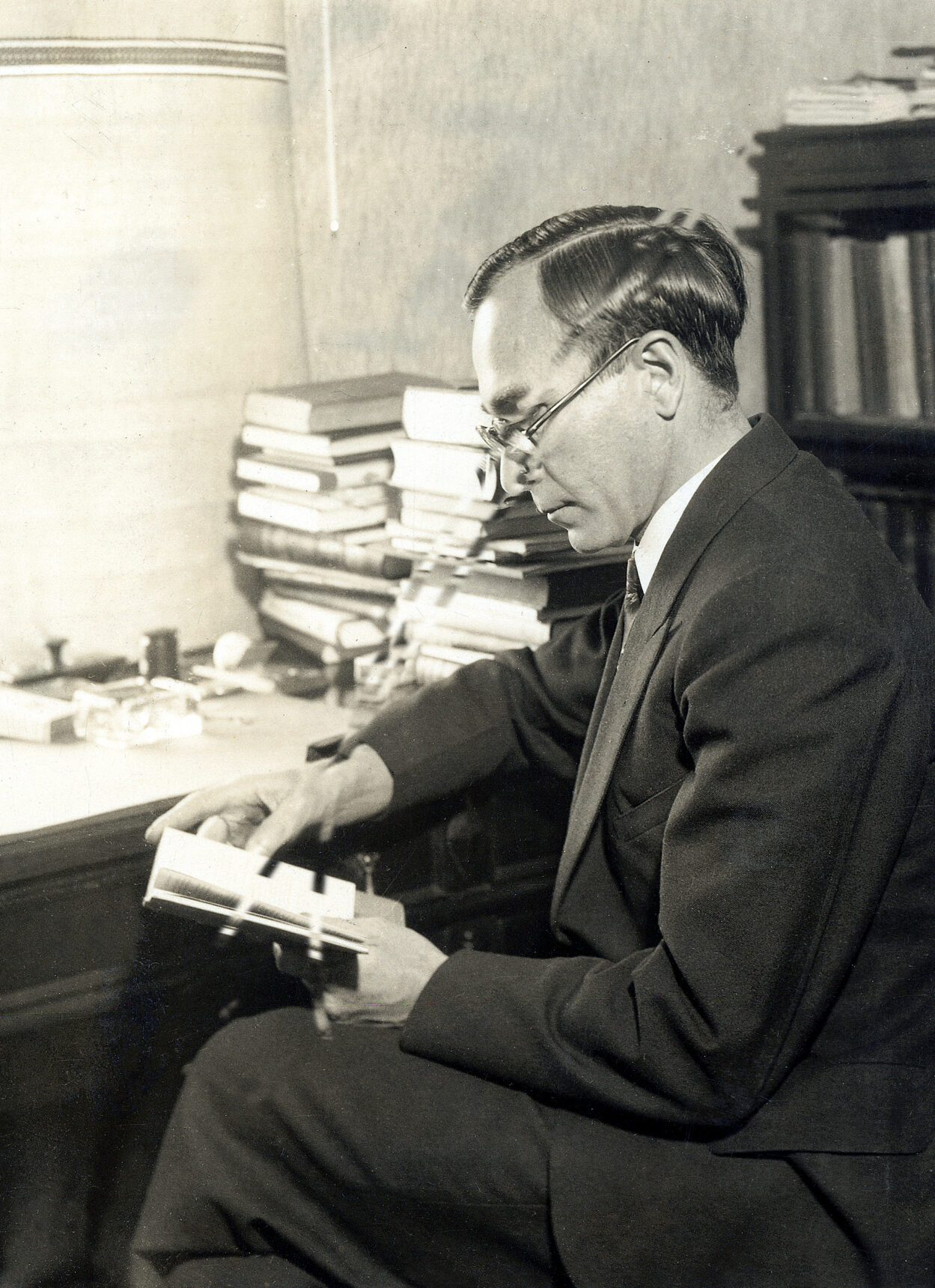December 2020

## How the Slowest Computer Programs Illuminate Math’s Fundamental Limits

### The goal of the “busy beaver” game is to find the longest-running computer program. Its pursuit has surprising connections to some of the most profound questions and concepts in mathematics.

#### By John Pavlus

Programmers normally want to minimize the time their code takes to execute. But in 1962, the Hungarian mathematician Tibor Radó posed the opposite problem. He asked: How long can a simple computer program possibly run before it terminates? Radó nicknamed these maximally inefficient but still functional programs “busy beavers.”

Finding these programs has been a fiendishly diverting puzzle for programmers and other mathematical hobbyists ever since it was popularized in Scientific American’s “Computer Recreations” column in 1984. But in the last several years, the busy beaver game, as it’s known, has become an object of study in its own right, because it has yielded connections to some of the loftiest concepts and open problems in mathematics.

“In math, there is a very permeable boundary between what’s an amusing recreation and what is actually important,” said Scott Aaronson, a theoretical computer scientist at the University of Texas, Austin who published a survey of progress in “BusyBeaverology.”

The recent work suggests that the search for long-running computer programs can illuminate the state of mathematical knowledge, and even tell us what’s knowable. According to researchers, the busy beaver game provides a concrete benchmark for evaluating the difficulty of certain problems, such as the unsolved Goldbach conjecture and Riemann hypothesis. It even offers a glimpse of where the logical bedrock underlying math breaks down. The logician Kurt Gödel proved the existence of such mathematical terra incognita nearly a century ago. But the busy beaver game can show where it actually lies on a number line, like an ancient map depicting the edge of the world.

An Uncomputable Computer Game

The busy beaver game is all about the behavior of Turing machines—the primitive, idealized computers conceived by Alan Turing in 1936. A Turing machine performs actions on an endless strip of tape divided into squares. It does so according to a list of rules. The first rule might say:

If the square contains a 0, replace it with a 1, move one square to the right and consult rule 2. If the square contains a 1, leave the 1, move one square to the left and consult rule 3.

Each rule has this forking choose-your-own-adventure style. Some rules say to jump back to previous rules; eventually there’s a rule containing an instruction to “halt.” Turing proved that this simple kind of computer is capable of performing any possible calculation, given the right instructions and enough time.

As Turing noted in 1936, in order to compute something, a Turing machine must eventually halt—it can’t get trapped in an infinite loop. But he also proved that there’s no reliable, repeatable method for distinguishing machines that halt from machines that simply run forever—a fact known as the halting problem.

The busy beaver game asks: Given a certain number of rules, what’s the maximum number of steps that a Turing machine can take before halting?Tibor Radó, shown here in an undated photo, invented the busy beaver game as a way of making the theoretical notion of uncomputability concrete. Courtesy of The Ohio State University Archives

For instance, if you’re only allowed one rule, and you want to ensure that the Turing machine halts, you’re forced to include the halt instruction right away. The busy beaver number of a one-rule machine, or BB(1), is therefore 1.

But adding just a few more rules instantly blows up the number of machines to consider. Of 6,561 possible machines with two rules, the one that runs the longest—six steps—before halting is the busy beaver. But some others simply run forever. None of these are the busy beaver, but how do you definitively rule them out? Turing proved that there’s no way to automatically tell whether a machine that runs for a thousand or a million steps won’t eventually terminate.

That’s why finding busy beavers is so hard. There’s no general approach for identifying the longest-running Turing machines with an arbitrary number of instructions; you have to puzzle out the specifics of each case on its own. In other words, the busy beaver game is, in general, “uncomputable.”

Proving that BB(2) = 6 and that BB(3) = 107 was difficult enough that Radó’s student Shen Lin earned a doctorate for the work in 1965. Radó considered BB(4) “entirely hopeless,” but the case was finally solved in 1983. Beyond that, the values virtually explode; researchers have identified a five-rule Turing machine, for instance, that runs for 47,176,870 steps before stopping, so BB(5) is at least that big. BB(6) is at least 7.4 × 1036,534.  Proving the exact values “will need new ideas and new insights, if it can be done at all,” said Aaronson.

Threshold of Unknowability

William Gasarch, a computer scientist at the University of Maryland, College Park, said he’s less intrigued by the prospect of pinning down busy beaver numbers than by “the general concept that it’s actually uncomputable.” He and other mathematicians are mainly interested in using the game as a yardstick for gauging the difficulty of important open problems in mathematics—or for figuring out what is mathematically knowable at all.

The Goldbach conjecture, for instance, asks whether every even integer greater than 2 is the sum of two primes. Proving the conjecture true or false would be an epochal event in number theory, allowing mathematicians to better understand the distribution of prime numbers. In 2015, an anonymous GitHub user named Code Golf Addict published code for a 27-rule Turing machine that halts if—and only if—the Goldbach conjecture is false. It works by counting upward through all even integers greater than 4; for each one, it grinds through all the possible ways to get that integer by adding two others, checking whether the pair is prime. When it finds a suitable pair of primes, it moves up to the next even integer and repeats the process. If it finds an even integer that can’t be summed by a pair of prime numbers, it halts.

Running this mindless machine isn’t a practical way to solve the conjecture, because we can’t know if it will ever halt until it does. But the busy beaver game sheds some light on the problem. If it were possible to compute BB(27), that would provide a ceiling on how long we’d have to wait for the Goldbach conjecture to be settled automatically. That’s because BB(27) corresponds to the maximum number of steps this 27-rule Turing machine would have to execute in order to halt (if it ever did). If we knew that number, we could run the Turing machine for exactly that many steps. If it halted by that point, we’d know the Goldbach conjecture was false. But if it went that many steps and didn’t halt, we’d know for certain that it never would—thus proving the conjecture true.

The rub is that BB(27) is such an incomprehensibly huge number that even writing it down, much less running the Goldbach-falsifying machine for that many steps, isn’t remotely possible in our physical universe. Nevertheless, that incomprehensibly huge number is still an exact figure whose magnitude, according to Aaronson, represents “a statement about our current knowledge” of number theory.

In 2016, Aaronson established a similar result in collaboration with Yuri Matiyasevich and Stefan O’Rear. They identified a 744-rule Turing machine that halts if and only if the Riemann hypothesis is false. The Riemann hypothesis also concerns the distribution of prime numbers and is one of the Clay Mathematics Institute’s “Millennium Problems” worth \$1 million. Aaronson’s machine will deliver an automatic solution in BB(744) steps. (It works by essentially the same mindless process as the Goldbach machine, iterating upward until it finds a counterexample.)

Of course, BB(744) is an even more unattainably large number than BB(27). But working to pin down something easier, like BB(5), “may actually turn up some new number theory questions that are interesting in their own right,” Aaronson said. For instance, the mathematician Pascal Michel proved in 1993 that the record-holding five-rule Turing machine exhibits behavior similar to that of the function described in the Collatz conjecture, another famous open problem in number theory.

“So much of math can be encoded as a question of, ‘Does this Turing machine halt or not?’” Aaronson said. “If you knew all the busy beaver numbers, then you could settle all of those questions.”

More recently, Aaronson has used a busy-beaver-derived yardstick to gauge what he calls “the threshold of unknowability” for entire systems of mathematics. Gödel’s famous incompleteness theorems of 1931 proved that any set of basic axioms that could serve as a possible logical foundation for mathematics is doomed to one of two fates: Either the axioms will be inconsistent, leading to contradictions (like proving that 0 = 1), or they’ll be incomplete, unable to prove some true statements about numbers (like the fact that 2 + 2 = 4). The axiomatic system underpinning almost all modern math, known as Zermelo-Fraenkel (ZF) set theory, has its own Gödelian boundaries—and Aaronson wanted to use the busy beaver game to establish where they are.

In 2016, he and his graduate student Adam Yedidia specified a 7,910-rule Turing machine that would only halt if ZF set theory is inconsistent. This means BB(7,910) is a calculation that eludes the axioms of ZF set theory. Those axioms can’t be used to prove that BB(7,910) represents one number instead of another, which is like not being able to prove that 2 + 2 = 4 instead of 5.

O’Rear subsequently devised a much simpler 748-rule machine that halts if ZF is inconsistent—essentially moving the threshold of unknowability closer, from BB(7,910) to BB(748). “That is a kind of a dramatic thing, that the number [of rules] is not completely ridiculous,” said Harvey Friedman, a mathematical logician and emeritus professor at Ohio State University. Friedman thinks that the number can be brought down even further: “I think maybe 50 is the right answer.” Aaronson suspects that the true threshold may be as close as BB(20).

Whether near or far, such thresholds of unknowability definitely exist. “This is the vision of the world that we have had since Gödel,” said Aaronson. “The busy beaver function is another way of making it concrete.”

Lead image: A visualization of the longest-running five-rule Turing machine currently known. Each column of pixels represents one step in the computation, moving from left to right. Black squares show where the machine has printed a 1. The far right column shows the state of the computation when the Turing machine halts. Credit: Quanta Magazine; source: Peter Krumins

Reprinted with permission from Quanta Magazine's Abstractions blog.